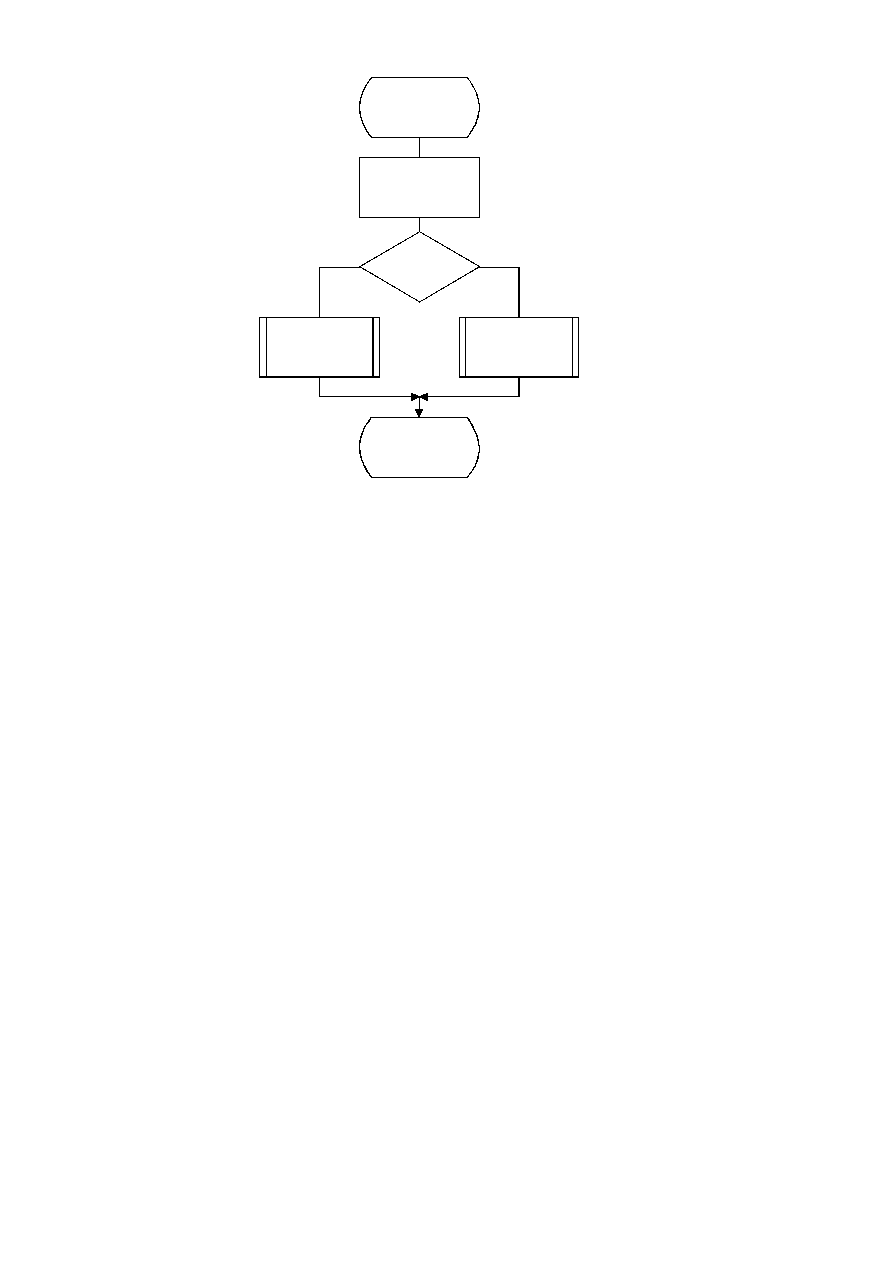ISO/IEC 10918-1 : 1993(E)
TISO1360-93/d074
Encode_DC_DIFF
V = DIFF
V = 0
?
No
Yes
Code_1(S0)
Encode_V(S0)
Code_0(S0)
Done
Figure F.4 ­ Coding model for arithmetic coding of DC difference
Figure F.4 [D74] = 11.5 cm = 449 %
The context-indices SE and S0 used in the AC coding procedures are defined in Table F.5 (see F.1.4.4.2). In Figure F.5,
K is the index to the zig-zag sequence position. For the sequential scan, Kmin is 1 and Se is 63. The V
=
0 decision is part
of a loop which codes runs of zero coefficients. Whenever the coefficient is non-zero, "Encode_V(S0)" codes the sign and
magnitude of the coefficient. Each time a non-zero coefficient is coded, it is followed by an EOB decision. If the EOB
occurs, a 1-decision is coded to indicate that the coding of the block is complete. If the coefficient for K
=
Se is not zero,
the EOB decision is skipped.
F.1.4.3
Encoding the binary decision sequence for non-zero DC differences and AC coefficients
Both the DC difference and the AC coefficients are represented as signed two's complement integer values. The
decomposition of these signed integer values into a binary decision tree is done in the same way for both the DC and AC
coding models.
Although the binary decision trees for this section of the DC and AC coding models are the same, the statistical models
for assigning statistics bins to the binary decisions in the tree are quite different.
F.1.4.3.1
Structure of the encoding decision sequence
The encoding sequence can be separated into three procedures, a procedure which encodes the sign, a second procedure
which identifies the magnitude category, and a third procedure which identifies precisely which magnitude occurred
within the category identified in the second procedure.
At the point where the binary decision sequence in Encode_V(S0) starts, the coefficient or difference has already been
determined to be non-zero. That determination was made in the procedures in Figures F.4 and F.5.
Denoting either DC differences (DIFF) or AC coefficients as V, the non-zero signed integer value of V is encoded by the
sequence shown in Figure F.6. This sequence first codes the sign of V. It then (after converting V to a magnitude and
decrementing it by 1 to give Sz) codes the magnitude category of Sz (code_log2_Sz), and then codes the low order
magnitude bits (code_Sz_bits) to identify the exact magnitude value.
94
CCITT Rec. T.81 (1992 E)